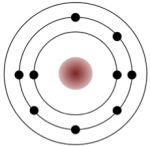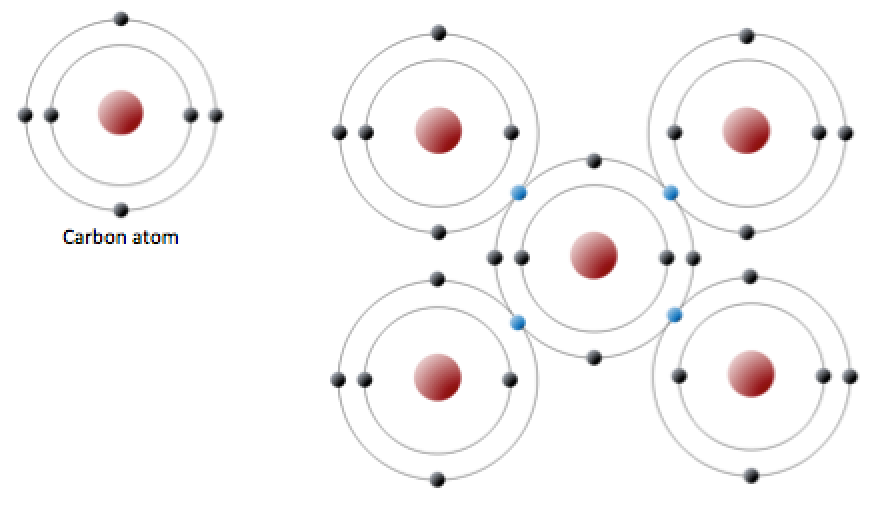# 2.2: Bonding and Lattices

$$\newcommand{\vecs}{\overset { \rightharpoonup} {\mathbf{#1}} }$$ $$\newcommand{\vecd}{\overset{-\!-\!\rightharpoonup}{\vphantom{a}\smash {#1}}}$$$$\newcommand{\id}{\mathrm{id}}$$ $$\newcommand{\Span}{\mathrm{span}}$$ $$\newcommand{\kernel}{\mathrm{null}\,}$$ $$\newcommand{\range}{\mathrm{range}\,}$$ $$\newcommand{\RealPart}{\mathrm{Re}}$$ $$\newcommand{\ImaginaryPart}{\mathrm{Im}}$$ $$\newcommand{\Argument}{\mathrm{Arg}}$$ $$\newcommand{\norm}{\| #1 \|}$$ $$\newcommand{\inner}{\langle #1, #2 \rangle}$$ $$\newcommand{\Span}{\mathrm{span}}$$ $$\newcommand{\id}{\mathrm{id}}$$ $$\newcommand{\Span}{\mathrm{span}}$$ $$\newcommand{\kernel}{\mathrm{null}\,}$$ $$\newcommand{\range}{\mathrm{range}\,}$$ $$\newcommand{\RealPart}{\mathrm{Re}}$$ $$\newcommand{\ImaginaryPart}{\mathrm{Im}}$$ $$\newcommand{\Argument}{\mathrm{Arg}}$$ $$\newcommand{\norm}{\| #1 \|}$$ $$\newcommand{\inner}{\langle #1, #2 \rangle}$$ $$\newcommand{\Span}{\mathrm{span}}$$$$\newcommand{\AA}{\unicode[.8,0]{x212B}}$$

As we’ve just seen, an atom seeks to have a full outer shell (i.e., eight electrons for most elements, or two electrons for hydrogen and helium) to be atomically stable. This is accomplished by transferring or sharing electrons with other atoms.HomeFigure $$\PageIndex{1}$$ A very simplified electron configuration of sodium and chlorine atoms (top). Sodium gives up an electron to become a cation (bottom left) and chlorine accepts an electron to become an anion (bottom right). 2.02: Bonding and Lattices#fig2.3

Sodium has 11 electrons: two in the first shell, eight in the second, and one in the third (Figure $$\PageIndex{1}$$). Sodium readily gives up that single third-shell electron, and when it loses this one negative charge, it becomes positively charged (because it now has 11 protons and only 10 electrons). By giving up its lone third-shell electron, sodium ends up with a full outer shell. Chlorine, on the other hand, has 17 electrons: two in the first shell, eight in the second, and seven in the third. Chlorine readily accepts an eighth electron to fill its third shell, and therefore becomes negatively charged because it has 17 protons and 18 electrons. In changing their number of electrons, these atoms become ions—the sodium loses an electron to become a positive ion or cation, and the chlorine gains an electron to become a negative ion or anion (Figure $$\PageIndex{1}$$).

Since negative and positive charges attract, sodium and chlorine ions can stick together, creating an ionic bond. Electrons can be thought of as being transferred from one atom to another in an ionic bond. Common table salt (NaCl) is a mineral composed of chlorine and sodium linked together by ionic bonds (Figure $$\PageIndex{1}$$). The mineral name for NaCl is halite.

An element like chlorine can also form bonds without forming ions. For example, two chlorine atoms, which each seek an eighth electron in their outer shell, can share an electron in what is known as a covalent bond to form chlorine gas (Cl2) (Figure $$\PageIndex{2}$$). Electrons are shared in a covalent bond.Figure $$\PageIndex{2}$$ Depiction of a covalent bond between two chlorine atoms. The electrons are black in the left atom and blue in the right atom. Two electrons are shared (one black and one blue) so that each atom “appears” to have a full outer shell.
##### Exercise 2.1 Cations, anions, and ionic bonding

A number of elements are listed below along with their atomic numbers. Assuming that the first electron shell can hold two electrons and subsequent electron shells can hold eight electrons, sketch in the electron configurations for these elements. Predict whether the element is likely to form a cation (+) or an anion (−) when electron transfer takes place, and what charge it would have (e.g., +1, +2, −1).

The first one is done for you. Fluorine needed an extra electron to have 8 in its outermost shell, and in gaining that electron it became negatively charged.

See Appendix 3 for Exercise 2.1 answers.

 Fluorine (9)anion (−1) Lithium (3)________ Magnesium (12)________ Argon (18)________ Chlorine (17)________ Beryllium (3)________ Oxygen (8)________ Sodium (11)________

A carbon atom has six protons and six electrons; two of the electrons are in the inner shell and four in the outer shell (Figure $$\PageIndex{3}$$). Carbon would need to gain or lose four electrons to have a filled outer shell, and this would create too great a charge imbalance for the ion to be stable. On the other hand, carbon can share electrons to create covalent bonds. In the mineral diamond, the carbon atoms are linked together in a three-dimensional framework, where each carbon atom is bonded to four other carbon atoms and every bond is a very strong covalent bond. In the mineral graphite, the carbon atoms are linked together in sheets or layers (Figure $$\PageIndex{3}$$), and each carbon atom is covalently bonded to three others. Graphite-based compounds, which are strong because of the strong intra-layer covalent bonding, are used in high-end sports equipment such as ultralight racing bicycles. Graphite itself is soft because the bonding between these layers is relatively weak, and it is used in a variety of applications, including lubricants and pencils.Figure $$\PageIndex{3}$$ The electron configuration of carbon (left) and the sharing of electrons in covalent bonding of diamond (right). The electrons shown in blue are shared between adjacent Carbon atoms. Although shown here in only two dimensions, diamond has a three-dimensional structure as shown on Figure $$\PageIndex{5}$$. [Image description]

Silicon and oxygen bond together to create a silica tetrahedron, which is a four-sided pyramid shape with O at each corner and Si in the middle (Figure $$\PageIndex{4}$$). This structure is the building block of the silicate minerals (which are described in Section 2.4). The bonds in a silica tetrahedron have some of the properties of covalent bonds and some of the properties of ionic bonds. As a result of the ionic character, silicon becomes a cation (with a charge of +4) and oxygen becomes an anion (with a charge of –2). The net charge of a silica tetrahedron (SiO4) is: 4 + 4(−2) = 4 − 8 = −4. As we will see later, silica tetrahedra (plural of tetrahedron) link together in a variety of ways to form most of the common minerals of the crust.Figure $$\PageIndex{4}$$ The silica tetrahedron, the building block of all silicate minerals. (Because the silicon ion has a charge of +4 and the four oxygen ions each have a charge of −2, the silica tetrahedron has a net charge of −4.)

Most minerals are characterized by ionic bonds, covalent bonds, or a combination of the two, but there are other types of bonds that are important in minerals, including metallic bonds and weaker electrostatic forces (hydrogen or Van der Waals bonds). Metallic elements have outer electrons that are relatively loosely held. (The metals are highlighted on the periodic table in Appendix 1.) When bonds between such atoms are formed, these electrons can move freely from one atom to another. A metal can thus be thought of as an array of positively charged atomic nuclei immersed in a sea of mobile electrons. This feature accounts for two very important properties of metals: their electrical conductivity and their malleability (they can be deformed and shaped).

Molecules that are bonded ionically or covalently can also have other weaker electrostatic forces holding them together. Examples of this are the force holding graphite sheets together and the attraction between water molecules.

What’s with all of these “sili” names?

The element silicon is one of the most important geological elements and is the second-most abundant element in Earth’s crust (after oxygen). Silicon bonds readily with oxygen to form a silica tetrahedron (Figure $$\PageIndex{4}$$). Pure silicon crystals (created in a lab) are used to make semi-conductive media for electronic devices. A silicate mineral is one in which silicon and oxygen are present as silica tetrahedra. Silica also refers to a chemical component of a rock and is expressed as % SiO2. The mineral quartz is made up entirely of silica tetrahedra, and some forms of quartz are also known as “silica”. Silicone is a synthetic product (e.g., silicone rubber, resin, or caulking) made from silicon-oxygen chains and various organic molecules. To help you keep the “sili” names straight, here is a summary table:

“Sili” name Definition
Table 2.3 Summary of “Sili” names
Silicon The 14th element
Silicon wafer A crystal of pure silicon sliced very thinly and used for electronics
Silica tetrahedron A combination of one silicon atom and four oxygen atoms that form a tetrahedron
% silica The proportion of a rock that is composed of the component SiO2
Silica A solid made out of SiO2 (but not necessarily a mineral – e.g., opal)
Silicate A mineral that contains silica tetrahedra (e.g., quartz, feldspar, mica, olivine)
Silicone A flexible synthetic material made up of Si–O chains with attached organic molecules

Elements that have a full outer shell are described as inert because they do not tend to react with other elements to form compounds. That’s because they don’t need to lose or gain any electrons to become stable, and so they don’t become ions. They all appear in the far-right column of the periodic table. Examples are: helium, neon, argon, etc.

As described in Chapter 1, all minerals are characterized by a specific three-dimensional pattern known as a lattice or crystal structure. These structures range from the simple cubic pattern of halite (NaCl) (Figure $$\PageIndex{1}$$), to the very complex patterns of some silicate minerals. Two minerals may have the same composition, but very different crystal structures and properties. Graphite and diamond, for example, are both composed only of carbon, but while diamond is the hardest substance known, graphite is softer than paper. Their lattice structures are compared in Figure $$\PageIndex{5}$$.Figure $$\PageIndex{5}$$ A depiction of the lattices of graphite and diamond.

Mineral lattices have important implications for mineral properties, as exemplified by the hardness of diamond and the softness of graphite. Lattices also determine the shape that mineral crystals grow in and how they break. For example, the right angles in the lattice of the mineral halite (Figure $$\PageIndex{1}$$) influence both the shape of its crystals (cubic), and the way those crystals break (Figure $$\PageIndex{6}$$).Figure $$\PageIndex{6}$$ Cubic crystals (left) and right-angle cleavage planes (right) of the mineral halite. If you look closely at the cleavage fragment on the right, you can see where it would break again (cleave) along a plane parallel to an existing surface. In most minerals, cleavage planes do not align with crystal surfaces.

## Image Descriptions

Figure $$\PageIndex{1}$$ image description: Sodium has one electron in its outer shell and chlorine has 7 electrons in it its outer shell. Sodium’s one outer electron goes to chlorine which makes Chlorine slightly negative and Sodium slightly positive. They attract each other and together they form Sodium Chloride. [Return to Figure $$\PageIndex{1}$$]

Figure $$\PageIndex{3}$$ image description: (Left) A carbon atom has two electrons in its inner shell and four electrons in its outer shell. (Right) One Carbon atom shares electrons with four other carbon atoms to form a complete outer shell. [Return to Figure $$\PageIndex{3}$$]

• Figure $$\PageIndex{6}$$ (left): Halite. © Rob Lavinsky, iRocks.com. CC BY-SA.